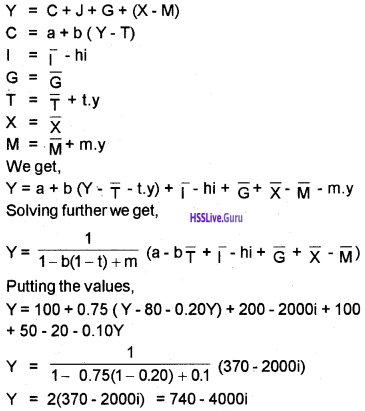# Plus Two Economics Chapter Wise Questions and Answers Chapter 6 Open Economy Macroeconomics

Students can Download Chapter 6 Open Economy Macroeconomics Questions and Answers, Plus Two Economics Chapter Wise Questions and Answers helps you to revise the complete Kerala State Syllabus and score more marks in your examinations

## Kerala Plus Two Economics Chapter Wise Questions and Answers Chapter 6 Open Economy Macroeconomics

### Plus Two Economics Open Economy Macroeconomics One Mark Questions and Answers

Question 1.
Name the ‘balancing item’ affecting the inability to record all international transactions accurately?
Errors and omissions

Question 2.
The amount of rupees required to buy one US\$ is known as ………….
(i) Rupee dollar exchange rate
(ii) Dollar rupee exchange rate
(iii) Real exchange rate
(iv) Real effective exchange rate
(i) Rupee dollar exchange rate

Question 3.
Which among the following is a component of BOP account?
(i) Current account
(ii) Capital account
(iii) Official reserve
(iv) All the above
(iv) All the above

Question 4.
WTO was formed in?
(i) 1948
(ii) 1964
(iii) 1991
(iv) 1995
(iv) 1995

Question 5.
If export > imports, it represents
(iv) None of these aboveQuestion 6.
Which of the following would be an appropriate policy to reduce a Balance of Payment / Deficit.
(i) An increase in government spending.
(ii) A cut in the level of indirect taxes
(iii) An increase in interest rates
(iv) A decrease in interest rates
(iii) An increase in interest rates

### Plus Two Economics Open Economy Macroeconomics Two Mark Questions and Answers

Question 1.
How is the exchange rate determined under flexible exchange rate regime?
In case of flexible exchange rates, the exchange rate changes to clear the market to equate the demand for and supply of foreign exchange.

Question 2.
List two items of the capital account of balance of payment account?

1. Gold movement
2. Reserve, Monetary gold & SDR

Question 3.
When will balance of trade shows a deficit?
Balance of trade shows a surplus when exports are greater than imports. That is, Surplus balance of trade = Exports > Imports

Question 4.
Name two sources of demand for foreign exchange.
Two sources of demand for foreign exchange are:

1. To purchase goods and services from abroad.
2. To send gifts and grants to foreign countries.

Question 5.
The value of a country’s import of goods is ₹200 crore and value of export of goods is ₹250 crore. Find out its balance of trade.
Balance of trade = Value of exports – value of imports = 250 – 200 = ₹50 crore

Question 6.
Identify the items to be included to trade balance to get current account balance.

2. Net transfers

Question 7.
Identify the situation mentioned below.

1. Rupee-dollar exchange rate change from 45 to 50.
2. Rupee-dollar exchange rate changed from 45 to 43.

1. Depreciation
2. AppreciationQuestion 8.
State the National Income identity for an open economy.
Y = C + IG + (X – M)
Where,

• Y = National Income
• C = Comsumption
• I = Investment
• G = Government spending
• X = Export
• M = Import

Question 9.
The consumption function and import function of an economy can be given as,
C = a + b.y
M = M + m.y Identity the letters

1. b
2. a
3. m

1. Marginal propensity to consume
2. Autonomous consumption
3. Marginal propensity to import

Question 10.
If MPC = 0.8, and increase in autonomous demand is 200, calculate multiplier and the national output.
MPC = b – c = 0.8
Therefore multiplier is
$$\begin{array}{l} {\frac{1}{1-C} \text { or } \frac{1}{1-b}} \\ {\text { i.e. } \frac{1}{1-0.8}=\frac{1}{0.2}} \\ {=5} \end{array}$$
National output is 5 × 200 = 1,000

Question 11.
Calculate the open economy multiplier if c = 0.5, m = 0.3. Increase in autonomous demand = 200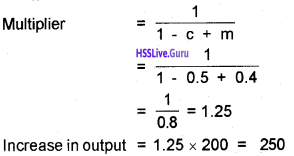Question 12.
What happens to the aggregate demand due to

1. A leakage from the circular flow of income
2. A injection to the circular flow of income

AD falls due to the leakage from circular flow of income and AD increases due to injection in too the circular flow of income.

Question 13.
List out the expert of services from the following……..
(a) India buys a new technology from France
(b) A Japaneese tourist visits India
(c) An Indian student registers for a UK exam
(d) an Indian doctor going to work in the US.
b and d are examples of expert services from India.

Question 14.
Analyse the effect of the following on imports and exchange rate.

1. Appreciation of domestic currency.
2. Depreciation of domestic currency
3. Increase in foreign direct investment.
4. Increase in import duty.

1. Increase in Imports, Fall in Exchange Rate
2. Decrease in Imports, Rise in Exchange Rate
3. Increase in Imports, Fall in Exchange Rate
4. Decrease in Imports, Fall in Exchange Rate

### Plus Two Economics Open Economy Macroeconomics Three Mark Questions and Answers

Question 1.
Classify the following into visible and invisible.
Steel, computer software, shipping services, wheat, machinery, food articles, banking, IT- enabled services, crude oil, shipping, textiles, Online business.

 Visibles Invisibles Steel Shipping Services Textiles Banking Wheat IT Enabled Services Machinery Shipping Food Articles Insurance Crude Oil Online Business

Question 2.
Classify the following into current account and capital account.
Foreign direct investment, borrowing from abroad, export earning from merchandise, export earnings from banking services, earning from tourism, foreign portfolio investment.

 Current Account Capital Account Export earnings from banking services Foreign direct investment Earning from tourism Borrowing from abroad Export earnings from merchandise Foreign portfolio investment

Question 3.
The open economy multiplier is smaller than that in a closed economy. Do you agree? Give reason.
Yes, I do agree with this statement.
The open economy multiplier is smaller than that in a closed economy because a part of domestic demand falls on foreign goods. An increase in autonomous demand thus leads to a smaller increase in output compared to a closed economy. It also results in a deterioration of the trade balanceQuestion 4.
Complete the following table.

• Export > Import
• Export = Import
• Export < Import

• Export > Import – Trade surplus
• Export = Import – Trade balance
• Export < Import – Trade deficit

Question 5.
Export promotion is one of the key factors for correcting disequilibrium in BOR Is there any other measure for correcting BOP? If yes, suggest 3 measures.
Measures to correct BOP disequilibrium,

• Increase in production
• Reduction in imports
• Encouraging foreign investment
• Promotion of exports

Question 6.
What is the MPM (Marginal propensity to import) When M = 60 + 0.67?
Marginal propensity to import (MPM) is the fraction of an additional currency of income spent on imports. The concept of MPM is same as the marginal propensity to consume (MPC). Thus, demand for imports is to depend on income and have an autonomous component.

Question 7.
Point out the items included in current account transactions of BOP.
The current account includes receipts and payments on account of:

1. export and import of goods and services
2. tourism services
3. foreign investment incomes and out payments
4. private transfer payments
5. inter-government transfer payments.

Question 8.
What is trade deficit? Calculate the trade deficit from the following data.Trade deficit is the difference between export of goods and import of goods in trade in goods in current account. It is the situation where import is greater than export.
Export of goods = 90,660
Import of goods = 1,20,364 – 90,660 = 29,704 croresQuestion 9.
a. Recently the government of UK decided to relax the visa norms to Indian visitors.
b. The government of India approved a purchase of weapon for Indian defence from rest of the world for an amount of 82000 crores

1. How does these decisions affect the demand for foreign exchange?
2. Analyse the consequences in the foreign exchange market with the help of a diagram.
(supply curve of foreign exchange remain the same)

1. Demand for foreign exchange increases

2. diagram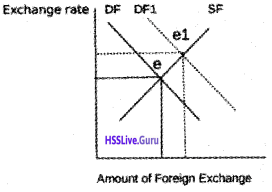### Plus Two Economics Open Economy Macroeconomics Five Mark Questions and Answers

Question 1.

1. tea, coir, tourism
2. rice, banking services, insurance service, transport services
3. foreign direct investment, foreign portfolio investment, remittances, borrowings
4. foreign investment, remittances, export earning from goods, export earning from services

1. Tourism. Others are visibles
2. Rice. Others are invisibles
3. Remittances. Others are capital receipts
4. Remittances. Others are capital receipts.

Question 2.
Match the following.

 A B Bretton Woods system 1944 SDR 1967 Fixed Exchange Rate Pegged Rate Triffin Dilemma Dollar accumulation Flexible Exchange Rate Floating Rate

 A B Bretton Woods system Pegged Rate SDR Dollar accumulation Fixed Exchange Rate 1944 Triffin Dilemma Floating Rate Flexible Exchange Rate 1967

Question 3.
If c (marginal propensity to consume) = 0.8 and m (marginal propensity to import) = 0.3,

1. Find the open and closed economy multiplier
2. If domestic autonomous demand increases by 100, find the output level in a closed and an open economy.

1. Closed economy multiplier
$$=\frac{1}{1-c}=\frac{1}{1-0.8}=\frac{1}{0.2}=5$$
Open economy multiplier
$$=\frac{1}{0.5}=\frac{1}{1-0.8+0.3}=\frac{1}{1-0.5}=\frac{1}{0.5}=2$$

2. If domestic autonomous demand increases by 100, in a closed economy output increases by 500 whereas it increases by only 200 in an open economy.Question 4.
Differentiate between fixed exchange rate and flexible exchange rate.
In a system of flexible exchange rates (also known as floating exchange rates), the exchange rate is determined by the forces of market demand and supply. Countries have had flexible exchange rate system ever since the breakdown of the Bretton Woods system in the early 1970s. Prior to that, most countries had fixed or what is called pegged exchange rate system, in which the exchange rate is pegged at a particular level.

Sometimes, a distinction is made between fixed and pegged exchange rates. Under a fixed exchange rate system, such as the gold standard, adjustment to BoP surpluses or deficits cannot be brought about through changes in the exchange rate.

Question 5.
What do you mean by managed floating? How far it is a mixture of fixed exchange rate and flexible exchange rates?
Without any formal international agreement, the world has moved on to what can be best described as a managed floating exchange rate system. It is a mixture of a flexible exchange rate system (the floating part) and a fixed rate system (the managed part).

Under this system, also called dirty floating, central banks intervene to buy and sell foreign currencies in an attempt to moderate exchange rate movements whenever they feel that such actions are appropriate. Official reserve transactions are, therefore, not equal to zero.

Question 6.
Distinguish between the nominal exchange rate and the real exchange rate. If you were to decide whether to buy domestic goods or foreign goods, which rate would be more relevant?
The price of one currency in terms of the other is known as the exchange rate. Nominal exchange rates are bilateral in the sense that they are exchange rates for one currency against another and they are nominal because they quote the exchange rate in money terms, i.e. so many rupees per dollar or per pound.

However, the real exchange rate is the ratio of foreign to domestic prices, measured in the same currency. It is defined as Real exchange rate = ePf/P where P and Pf are the price levels here and abroad, respectively, and e is the rupee price of foreign exchange (the nominal exchange rate).

The real exchange rate is often taken as a measure of a country’s international competitiveness. Therefore, real exchange rate is considered to be more relevant.

Question 7.
Balance of payment is a broader concept than balance of trade. Give explanation to this view.
Balance of trade is the record of a country’s visible export and visible imports. It includes only visible trade and excludes invisible trade of services. However, balance of payment is a more comprehensive term which denoted a country’s total monetary transactions with the rest of the world. It includes both visible and invisible trade of goods and, services.

The balance of payments (BoP) records the transactions in goods, services and assets between residents of a country with the rest of the world. There are two main accounts in the BoP the current account and the capital account.

Question 8.
The current account is differentiated from capital account. Do you agree? Give explanation.
Yes. The current account balance is the sum of the balance of merchandise trade, services and net transfers received from the rest of the world. The capital account balance is equal to capital flows from the rest of the world, minus capital flows to the rest of the world.Question 9.
Illustrate the method of determining equilibrium under flexible exchange rate system. Also, show the effect of increase in demand for imports in the foreign exchange markets.
In a system of flexible exchange rates, the exchange rate is determined by the forces of market demand and supply. In this case of flexible exchange rates without central bank intervention, the exchange rate moves to clear the market, to equate the demand for and supply of foreign exchange. In the following figure equilibrium exchange rate is e* which is determined by the forces of demand and supply.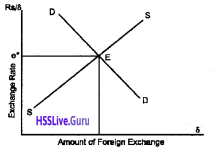At the initial equilibrium exchange rate e*, suppose there is now an excess demand for foreign exchange. To clear the market, the exchange rate must rise to the equilibrium value e1 as shown in the following figure.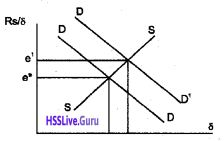The rise in exchange rate (depreciation) will cause the quantity of import demand to fall since the rupee price of imported goods rises with the exchange rate. Also, the quantity of exports demanded will increase since the rise in the exchange rate makes exports. less expensive to foreigners. At the new equilibrium, the supply and demand for foreign exchange is again equal.

Question 10.
Differentiate between devaluation and depreciation.
Devaluation means increase in exchange rate. Devaluation is said to occur when the exchange rate is increased by social action under a pegged exchange rate system. Devaluation is used as a tool to bridge the gap of trade deficit.

On the other hand, change in the price of foreign exchange under flexible exchange rate, when it becomes cheaper as compared to domestic currency is known as depreciation.

Question 11.
Compare balance of trade (BOT) and balance of payments (BOP).
Balance of trade is the difference between money value of imports and exports of material goods only whereas BOP is the difference between a country’s receipts and payments in foreign exchange. The difference between the two can be summarized as follows:

 BOT BOP 1. It records only merch­andise transactions 1. It records transactions relating to both goods and services 2. It does not record trans­actions of special nature. 2. It records transactions of capital nature. 3. It is a narrow concept because it is only one part of BOP account 3. It is wider concept because it includes balance of trade, balance of Services, balance of unrequired transfers and balance of capital transactions. 4. It may be favorable, un favorable or equilibrium 4. It always remains in balance in accounting sense because receipt side is always made to be equal to payment side

Question 12.
Complete the following flow chart.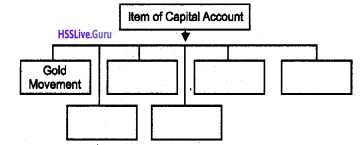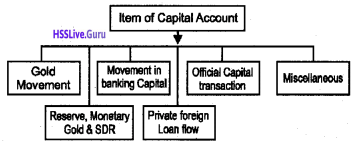Question 13.
Distinguish between autonomous and accommodating transactions?
International economic transactions are called autonomous when transactions are made independently of the state of the BOP. These items are called above the line.

On the other hand, accommodating transactions are determined by the net consequences of the autonomous items, that is whether the BOP is in surplus or deficit. These items are called ‘below the line.Question 14.
Suppose the equilibrium exchange rate is shown in the figure. What happens to this equilibrium situation when there is increase in demand for foreign exchange?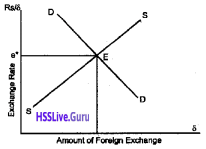When the demand for foreign exchange increases, there is rise in exchange rate (depreciation). At the higher exchange rate, more quantity of foreign exchange will be transacted. This is shown below.Question 15.
Distinguish between appreciation and depreciation. Identify what happens to the exchange rate of rupees in 2015 compared to 2014.

 Year Rupee dollar exchange rate 2014 50. 2015 60.

Appreciation refers to the increase the exchange rate of a currency. Depreciation refers to the decrease in the rate of exchange of currency. Both appreciation depreciation of exchange rate occurs due to the changes in the supply and demand of currencies. Compared to 2014 there is a depreciation of currency exchange rate in 2015.

Question 16.
The diagram below shows how the rate of exchange is determined in a free market.Show the effect of the following on the exchange rate.

1. The rate of interest of the country increases.
2. The rate of inflation of the nearby countries.

1. When the rate of interest increases the rate of exchange will increase. This is because an increased rate of interest would attract more depositors into the country, the demand for the currency would increase and the rate of interest also will increase as shown in the diagram below.2. When the inflation of the nearby countries increases the people around would prefer to buy goods from this country. So the demand for the currency would increase leading to an increase in the rate of exchange.Question 17.
Exchange rate is determined through different methods. Diagrams related with exchange rate are given below.1. Identify the Exchange rate system corresponding to each diagram
2. Distinguish between the two.

1. Diagram A is Flexible Exchange Rate System and Diagram B is Fixed Exchange Rate System

2. In a system of flexible exchange rates (also known as floating exchange rates), the exchange rate is determined by the forces of market demand and supply. Countries have had flexible exchange rate system ever since the breakdown of the Bretton Woods system in the early 1970s.

Prior to that, most countries had fixed or what is called pegged exchange rate system, in which the exchange rate is pegged at a particular level. Sometimes, a distinction is made between fixed and pegged exchange rates. Under a fixed exchange rate system, such as the gold standard, adjustment to BoP surpluses or deficits cannot be brought about through changes in the exchange rate.

### Plus Two Economics Open Economy Macroeconomics Eight Mark Questions and Answers

Question 1.
Determine the equilibrium level of income based on the following information.
C = 100 + 0.75 (Y – T)
I = 200 – 2000;
G = 100
T = 80 + 0.20Y
X = 50
M = 20 + 0.10Y